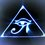# Absolute Value

Absolute Value is the magnitude of a number without regard to its sign.

In other words to take the absolute value of a number is to measure its distance from zero. Because distance is a positive measurement of course the values will be positive but this is in regards to distance and not direction. (In this sense absolute value is to relative value as speed is to velocity.)

If we have a number line:

$-10---------0---------+10$

The values of -10 and +10 are both 10 units from 0, although -10 is to the left and +10 is to the right.

Using a number line gives a good visual for absolute value but can be time consuming. A more practical method to calculate absolute values would be squaring the number and then taking the square root. This works because squaring a negative will result in a positive value. Thus when you try to revert back to the root of the square, you cannot. You can only determine its absolute value. Yes, simply changing the sign to positive would also work.

Find the absolute value of -11

$(-11)^{2} = 121$

$\sqrt{121} = 11$

This is my personal method for calculating absolute value. Although I must say it is a slippery slope and is too be used wisely because it doesn't work for many absolute value problems. Although it can be helpful at times such as inputting a formula for absolute value into a graphing calculator.Note by Brody Acquilano
6 years, 7 months ago

This discussion board is a place to discuss our Daily Challenges and the math and science related to those challenges. Explanations are more than just a solution — they should explain the steps and thinking strategies that you used to obtain the solution. Comments should further the discussion of math and science.

When posting on Brilliant:

• Use the emojis to react to an explanation, whether you're congratulating a job well done , or just really confused .
• Ask specific questions about the challenge or the steps in somebody's explanation. Well-posed questions can add a lot to the discussion, but posting "I don't understand!" doesn't help anyone.
• Try to contribute something new to the discussion, whether it is an extension, generalization or other idea related to the challenge.

MarkdownAppears as
*italics* or _italics_ italics
**bold** or __bold__ bold
- bulleted- list
• bulleted
• list
1. numbered2. list
1. numbered
2. list
Note: you must add a full line of space before and after lists for them to show up correctly
paragraph 1paragraph 2

paragraph 1

paragraph 2

[example link](https://brilliant.org)example link
> This is a quote
This is a quote
    # I indented these lines
# 4 spaces, and now they show
# up as a code block.

print "hello world"
# I indented these lines
# 4 spaces, and now they show
# up as a code block.

print "hello world"
MathAppears as
Remember to wrap math in $$ ... $$ or $ ... $ to ensure proper formatting.
2 \times 3 $2 \times 3$
2^{34} $2^{34}$
a_{i-1} $a_{i-1}$
\frac{2}{3} $\frac{2}{3}$
\sqrt{2} $\sqrt{2}$
\sum_{i=1}^3 $\sum_{i=1}^3$
\sin \theta $\sin \theta$
\boxed{123} $\boxed{123}$